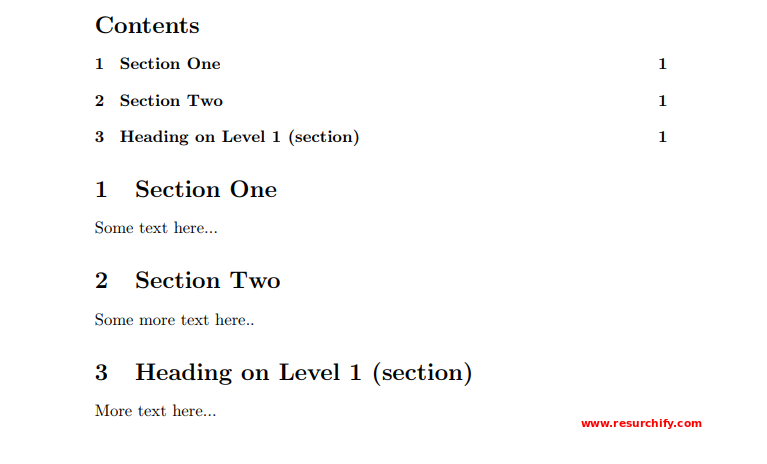Resurchify

Page Numbering in LaTeX

Page Numbering might not seem to be a big task. But it definitely helps a lot in bringing an official touch to the documents.

By default, the Page numbering in LaTeX uses Arabic numbers. We can change this to Roman numerals and/or letters. Also, multiple numbering styles can be combined in a single document. There is also a property where multiple numbering styles can be combined in a single document in LaTeX.

Below are the topics that we will be covering in this article.

Introduction

Let us go through an example to understand the basics of Page Numbering.

 1 2 3 4 5 6 7 8 9 10 11 12 \documentclass{article} \usepackage[utf8]{inputenc}   \pagenumbering{roman}   \begin{document}   \section{First section} ...   \end{document}

In the above example, we see that command \pagenumbering{roman} sets the page numbering to lowercase Roman numerals.

Below is the output obtained on running the above piece of code.Numbering styles in LaTeX

In LaTeX, we have several numbering styles. Following is the list of styles for Page numbering:

• alph: lowercase letters
• Alph: uppercase letters
• arabic: arabic numerals
• roman: lowercase roman numerals
• Roman: uppercase roman numerals

For example, in the above code, the lower-case Roman numerals were used.

Let us now have a look at another numbering style with the help of an example.

 1 2 3 4 5 6 7 8 9 10 11 12 \documentclass{article} \usepackage[utf8]{inputenc}   \pagenumbering{alph}   \begin{document}   \section{Test section} ...   \end{document}\pagenumbering{num_style} sets the layout of the page number.

Use of two page numbering styles in a single LaTeX document

In LaTeX, it is possible to add a combination of numbering style. For instance, in the document class book, there are two commands that help putting up Roman as well as Arabic numbers in a single document. Let us look at an example to understand this.

 1 2 3 4 5 6 7 8 9 10 11 12 13 14 15 16 17 18 19 20 21 22 \documentclass{book} \usepackage[utf8]{inputenc}   \begin{document}   \frontmatter   \addcontentsline{toc}{chapter}{Foreword} \Some text...   \addcontentsline{toc}{chapter}{Acknowledgement} Some text...   \tableofcontents   \mainmatter   \chapter{Chapter One}   This is the first chapter...   \end{document}Through the above example, we can understand that the commands that control the page numbering are:

\frontmatter: Starting from this command till \mainmatter command, Roman numerals will be used for numbering. This numbering appears in lowercase Roman numerals.

\mainmatter: This command restarts the page counter and changes the style to Arabic numbers.

If your document class is not book or you need more control over the page counter and the numbering style, see the next example:

 1 2 3 4 5 6 7 8 9 10 11 12 13 14 15 16 17 18 19 20 21 22 \documentclass{article} \usepackage[utf8]{inputenc} \usepackage[english]{babel}   \pagenumbering{roman}   \begin{document} \tableofcontents   \section{Section One} \setcounter{page}{4}   Some text here...   \section{Section Two} Some more text here..   \section{Heading on Level 1 (section)} \pagenumbering{arabic}   More text here... \end{document}Let us understand the different commands used in the above example.

In this example, we are explicitly mentioning the numbering styles and also, setting the counter to a specific number. Below is the explanation of the commands used.

• \pagenumbering{roman}: This command sets the page numbers to lowercase Roman numerals.
• \setcounter{page}{4}: Through this command, we set the page counter to 3 manually. All the subsequent pages are numbered starting the count from this number.
• \pagenumbering{arabic}: The page numbering is set to Arabic. When this happens, counter is restarted.

How to customize numbering styles in LaTeX

We can customize the page numbering style in LateX by using the package fancyhdr. For example, if you want to put the current page number in the context of the page numbers in the whole document (1 of 120, for instance), following steps can be followed to implement that.

 1 2 3 4 5 6 7 8 9 10 11 12 13 14 15 16 17 18 19 20 21 22 \documentclass{article} \usepackage[utf8]{inputenc} \usepackage[english]{babel} \usepackage{fancyhdr} \usepackage{lastpage}   \pagestyle{fancy} \fancyhf{}   \rfoot{Page \thepage \hspace{1pt} of \pageref{LastPage}}   \begin{document}   \tableofcontents   \section{Section One} Some text...   \section{Section Two} More text...   \end{document}Here, the package lastpage is imported by \usepackage{lastpage} so that we can refer the last page of the document. Then we set fancyhdr to display Page n of All" on the down right corner of the page, where n is the current page and All is the last page or total number of pages.

Conclusion

In this tutorial, we have learned how to add page number in LaTeX. Apart from this, we have seen how to change the page numbering style and also create combinations of numbering styles.